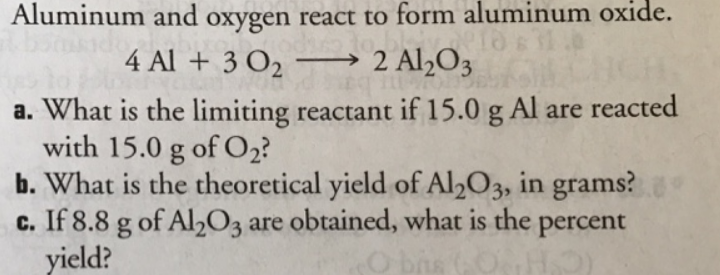# Problem: Aluminum and oxygen react to form aluminum oxide. 4 Al + 3 O2 --&gt;2 Al2O3a. What is the limiting reactant if 15.0 g Al are reacted with 15.0 g of O2?b. What is the theoretical yield of Al2O3, in grams?c. If 8.8 g of Al2O3 are obtained, what is the percent yield?

###### FREE Expert Solution91% (60 ratings)###### Problem Details

Aluminum and oxygen react to form aluminum oxide. 4 Al + 3 O2 -->2 Al2O3
a. What is the limiting reactant if 15.0 g Al are reacted with 15.0 g of O2?
b. What is the theoretical yield of Al2O3, in grams?
c. If 8.8 g of Al2O3 are obtained, what is the percent yield?